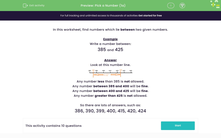# Find a number in the range 100 - 299

In this worksheet, students write a number between two given numbers.Key stage:  KS 2

Curriculum topic:   Number: Number and Place Value

Curriculum subtopic:   Order/Compare Numbers to 1000

Difficulty level:#### Worksheet Overview

In this activity, we will be finding numbers that lie between two given numbers.

A number line can help us work out which numbers would be between two numbers.

This number line could help us to find numbers between 185 and 225.Any number less than 185 is not allowed.

Any number between 185 and 225 will be fine.

Any number greater than 225 is not allowed.

So there are lots of possible answers, such as:

186, 195, 201, 213

Let's try an example question together.

Example

Find four numbers between 155 and 195.

Any number less than 555 is not allowed.

Any number between 155 and 195 will be fine.

Any number greater than 195 is not allowed.

There are many possible answers but some of them could include: 162, 173, 159, and 190.

Now it's your turn to try some questions.

### What is EdPlace?

We're your National Curriculum aligned online education content provider helping each child succeed in English, maths and science from year 1 to GCSE. With an EdPlace account you’ll be able to track and measure progress, helping each child achieve their best. We build confidence and attainment by personalising each child’s learning at a level that suits them.

Get started